# Solomonoff induction

Solomonoff induction is an idealized method of sequence prediction.

## Model selection vs sequence prediction

This section should answer the question of "what do we even mean by 'induction'?", "what does it mean to do good epistemology?" In all cases, we get some sort of data, and then we're supposed to make some prediction, but there are several possibilities for the nature of the prediction (are we predicting what we will likely see next? are we predicting what we think is the laws of physics? are we going to get an "x" value and asked to predict a "y" value that corresponds to it, like in supervised learning?). Each of these different "nature of the prediction" leads to a conceptually different form of induction:

Solomonoff induction is formulated in terms of sequence prediction so the nature of the prediction = the nature of the data, i.e. we want to predict the likely data we will observe next.

The other thing to mention is that the other kinds of "induction" can be converted into a sequence prediction problem or can be obtained by slightly modifying the Solomonoff induction scheme. This means that if we figure out a way to "do good sequence prediction", we will have essentially solved how to "do good induction"/"do good epistemology". (?) (I think this is right, but I am fuzzy on the details)

## Variants

(Note: I'm splitting off most of this to Variants of Solomonoff induction while keeping a high-level summary here)

There are many variants of Solomonoff induction that seem to all turn out essentially the same, but most resources on Solomonoff induction only talk about one or two of the variants, which makes it pretty confusing for someone trying to learn about it. It's also not obvious that all of these variants are essentially the same, so showing that would be nice.

Comparison dimensions:

• Determinism: are your programs probabilistic or deterministic? If deterministic, I think the programs require random coinflips as input.
• Kind of Turing machine used: ordinary, prefix, monotone
• Prediction length: finite vs infinite sequences
• Input (to UTM) length: finite vs infinite
• Solomonoff prior vs universal mixture; for universal mixture, you need to also specify the class over which you're mixing, and what you are summing over (programs, as in Eliezer's Arbital dialogue, or semimeasures, as in Legg's paper)
• Discrete vs continuous? (is this the same as finite vs infinite?)

List of variants considered in various resources:

• Li and Vitanyi:
•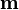$\mathbf m$ (defined in theorem 4.3.1 and example 4.3.3) seems like a discrete universal mixture. Input is finite. The "programs" (lower semicomputable discrete semimeasures$P_j$) are probabilistic.
• universal a priori probability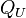$Q_U$ (definition 4.3.5): deterministic prefix machine, random coinflips as input, finite input (program), finite output
•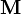$\mathbf M$
• universal a priori probability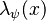$\lambda_\psi(x)$ (definition 4.5.6, p. 302)
•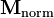$\mathbf{M}_{\mathrm{norm}}$ (a version of$\mathbf M$ that is a measure)
• Marcus Hutter's papers...
• Herrmann's "An Introduction to Solomonoff Induction With an Application to Scientific Confirmation Theory":
• Logical Induction Arbital page:
• deterministic, regular (rather than prefix-free) Turing machines

## Type of hypothesis

What is the type of a hypothesis? The type varies by formalization. For example:

• In classical statistical inference, a hypothesis is a constant that happens to be unknown, so e.g. it is a real number. This hypothesis then induces a probability distribution (called a "model").
• In Bayesian statistical inference, a hypothesis is an event (i.e. a subset of the sample space). There is a random variable representing the hypothesis (e.g.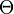$\Theta$), and you can get each individual hypothesis (e.g.$H_1 \subseteq \Omega$) by setting it to different values (e.g.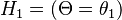$H_1 = (\Theta = \theta_1)$).
• In statistical learning (e.g. supervised learning), a hypothesis is some function$h : \mathcal X \to \mathcal Y$ from some set of objects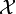$\mathcal X$ that we wish to label, to another set$\mathcal Y$ of labels (often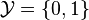$\mathcal Y = \{0,1\}$).
• In Solomonoff induction a hypothesis is a program (?) whose type depends on the variant of Solomonoff induction being used. In one of the versions, a hypothesis is a program that outputs bits; in another version a hypothesis assigns probabilities to bits.
• logical induction: this framework has various "characters" in it, namely a market, a deductive process, and traders. I guess a hypothesis can correspond to traders, who (unlike the other frameworks) only need to focus on a single pattern to try to exploit the market.

## Significance of random coin flips

 (p. 4)

"""The prior AIXI is based on is the Solomonoff prior. It is defined as the output of a universal Turing machine (UTM) whose inputs are coin-flips.

In other words, feed a UTM a random program. Normally, you’d think of a UTM as only being able to simulate deterministic machines. Here, however, the initial inputs can instruct the UTM to use the rest of the infinite input tape as a source of randomness to simulate a stochastic Turing machine.

Combining this with the previous idea about viewing Bayesian learning as a way of allocating “trust” to “experts” which meets a bounded loss condition, we can see the Solomonoff prior as a kind of ideal machine learning algorithm which can learn to act like any algorithm you might come up with, no matter how clever.""" -- one thing that's not clear to me with this interpretation is what if we want something to have probability 1/3? we can approximate it arbitrarily well using coinflips, but we can never get it exactly. contrast this to probabilistic programs, which can output 1/3 exactly.

"""(This trick is well known. For example, imagine that you have a fair coin and you need to simulate the coin that has probabilities 2/3 and 1/3. Then you generate a random real uniformly distributed in [0, 1] (by fair coin tossing) and compare this real number with threshold 2/3. To simulate the second coin tossing, you divide both intervals [0,2/3] and [2/3,1] in the same proportion 2 : 1. The algorithm described earlier does exactly this.)

Theorem 76 shows that it is enough to have a physical generator of independent symmetric random bits (a fair coin) to emulate arbitrary other computable probability distribution and even arbitrary continuous semimeasure.""" (Kolmogorov Complexity and Algorithmic Randomness, Shen et al., p. 120/134PDF)

See page 12 of : """In$M(hr)$, the random number,$r$, is used to make the probabilistic choices in string generation by the grammar described by$h$.

The expression$M(hr)$ is extremely interesting. The argument$hr$ consists of a random part preceded by a non-random description of a language. What would happen if the argument of$M$ was purely random: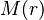$M(r)$?"""

"Probabilistic machines. A slightly different alternative interpretation views a monotone machine on random input as a probabilistic machine that has an internal random-bit generator to aid its computations. In particular, a monotone machine as defined above is a probabilistic machine that computes without any input, but with the possibility of using self-generated random bits. The value$\lambda_M(\mathbf{y})$ is then [interpreted] as the probability that$M$ in the course of its computation generates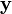$\mathbf y$. (See Shen et al., 20xx.)" ("Universal Prediction", Tom F. Sterkenburg, p. 67)

## Measures vs semimeasures

Multiple ways to think about semimeasures:

• "defective" measures that can be patched up by adding an extra element
• probability distribution over finite and infinite strings -- the "semi" part comes because of the inclusion of the finite strings. If all the finite strings had probability 0, then we would have a measure.

## Computable, computably enumerable, lower semicomputable, upper semicomputable

there is "computable" in the recursion theory sense, and then there is "computable" in the lower and upper approximations sense. I think these are equivalent; the latter is just trying to define the former in the case where the domain/range are uncountable.

## Turing machines, prefix machines, monotone machines

Kraft's inequality

prefix machine = prefix-free machine? (I think so)

Consider a prefix machine T. Then T(p)=x means that p is a self-delimiting program, and halts with output x. This means that T(p01010)!=x, since p01010 is not self-delimiting (because T halts immediately after reading p, so it doesn't get to the extra 01010 part). Like the monotone case, this is notationally a little confusing for me. I would rather have T(p) mean a separate expression, meaning if we feed T with p, then the output of that (if it ever halts). So in my world, T(p01010) is x, and x=x. Now the problem with this is that when we're summing over these programs, we need to filter out the non-self-delimiting ones. So for that we can just define some set like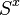$S^x$ (set of all p which are self-delimiting for x). I think this makes more sense, and is actually what the LW wiki page does, but not what marcus hutter does. I guess the way to think about this in terms of the hutter way is to say that if p01010 is on the tape then we never read past p, so it's not quite true to say T(p01010)=x. Instead, we should say T(p)=x. There is no way to feed T with p01010, since it refuses to read past p. So we should summarize this by saying that T(p01010) is undefined.

One difference between prefix machines and monotone machines: for prefix machines, there is no difference between saying "self-delimiting program" and "self-delimiting program for x". But for monotone machines, saying "minimal program" and "minimal program for x" are different. Actually, saying "minimal program" might not even make sense.

"can be computed by a monotone machine" = "can be computed by an interactive algorithm" = "process" (see Hay 2007)

In terms of notation, some authors use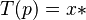$T(p) = x*$ and others use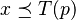$x \preceq T(p)$.

prefix machines are used for discrete version, and monotone machines are used for continuous version?

http://www.hutter1.net/ai/sintro2kc.pdf -- this is the only page that really makes the prefix vs montone distinction clear. For prefix we care that the machine halts, but for monotone we don't.

What functions can prefix/monotone machines compute? Can they compute everything a regular Turing machine can, but just add an "interactive" component? Or is there some Turing-computable function that prefix/monotone machines can't compute? -- since the work tape is bidirectional and we can move freely on it, we can start by copying the program to it, then going back to the leftmost nonblank square, then executing the program like a normal Turing machine, and finally copying the resulting tape onto the write-only output tape. This only works if the program is finite though.

Suppose the set of input programs is prefix-free. Then if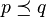$p \preceq q$, we must have$p=q$, so we automatically get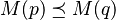$M(p) \preceq M(q)$. So prefix-free implies monotonicity.

Hutter says (p. 11) that "For given$x$,$\{p : T(p) = x*\}$ forms a prefix code". At first I was confused about this. What if a program prints "+" each time it reads a 0 and does nothing when it reads a 1? Then on input "000" it prints "+++", but if the input was "0001", then it seems like this would also print "+++", so the set of programs that print "+++" don't form a prefix code (because "000" is a prefix of "0001")! But looking more closely, Hutter says that$T(p) = x*$ means "$p$ is to the left of the input head when the last bit of$x$ is output". When the last bit of "+++" is output, the thing to the left of the input head is "000". So by this definition, it seems like "0001" will not be in the set of programs, i.e. we cannot say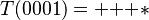$T(0001) = {+}{+}{+}*$. This is somewhat unintuitive to me (I wouldn't have expected this from the notation), but at least now it makes sense to say that the set of programs for a given output$x$ forms a prefix code.

So why do we sometimes use a prefix machine and sometimes use a monotone machine? If we're dealing with finite output strings, then we can require that the machine halts. So then program itself contains the halting instruction. But when we're dealing with infinite output strings, we also want to allow for the fact that maybe the machine won't halt, and that that's okay too. But if we allow non-halting programs, then we need to be more careful about which programs to allow.

I have an intuition that says if we take monotone machines but restrict to finite output, then we get exactly prefix machines.

consider program p:

print("+")
halt()

and program p':

print("+")
print("")
halt()

because of the halting instruction, p is not a prefix of p'. But if we're working with infinite programs q:

print("+")

and q':

print("+")
print("")

then q is a prefix of q'. Monotone machines prevent this kind of thing by saying that you're only allowed to call yourself is a program if you've just finished printing a non-empty string.

What if we take the set of all monotone programs, and insert halt() at every possible location?

## Two different ways to think about conditional predictions

Let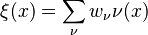$\xi(x) = \sum_\nu w_\nu \nu(x)$ be the universal mixture, where the$\nu$ are the hypotheses, and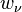$w_\nu$ is the prior weight placed on hypothesis$\nu$.

To find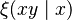$\xi(xy \mid x)$ we can use bayes to get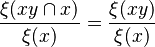$\frac{\xi(xy \cap x)}{\xi(x)} = \frac{\xi(xy)}{\xi(x)}$.

but we can also think about this as updating our belief in the experts/hypotheses. Given that x happened, some of our experts predicted that this would happen, so we should put more weight on what they say next (and similarly, some of our experts said this wouldn't happen, so we should be more skeptical of what they say next).

Our conditional weight on expert$\nu$, given that$x$ happened, is$w_\nu(x) = P(\nu\mid x) = \frac{P(x \mid \nu)P(\nu)}{P(x)} = \frac{\nu(x)w_\nu}{\xi(x)}$.

Now using these new weights, to predict xy, we should have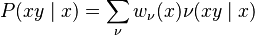$P(xy \mid x) = \sum_\nu w_\nu(x)\nu(xy \mid x)$.

Simplifying this, we get$\sum_\nu \frac{\nu(x)w_\nu}{\xi(x)} \cdot \frac{\nu(xy)}{\nu(x)} = \frac{\sum_\nu w_\nu\nu(xy)}{\xi(x)} = \frac{\xi(xy)}{\xi(x)} = \xi(xy\mid x)$, which is exactly what we had before!

So we can think of conditional sequence prediction in two different ways: (1) we could start out with an all-powerful prior, and just do regular bayesian updating using this prior; (2) given new evidence, we can update our beliefs in the experts, and then let those experts make conditional predictions, and finally aggregate those predictions.

## Applications

• there are some standard applications you can find in standard references
• AIXI
• carl's analogy to psychophysical laws -- rob bensinger's post about AIXI also talks about "qualia factory"
• malign prior stuff
• comparison to logical induction

## Questions/things to explain

• https://www.greaterwrong.com/posts/3xnkw6JkQdwc8Cfcf/is-the-human-brain-a-valid-choice-for-the-universal-turing
• i think stuart armstrong had a LW post related to this, about how you can be convinced that you've won the lottery based on what seems like a very small amount of data, but that actually, one has accumulated a bunch of "background data" prior to the final piece of data.
• incorporating actions/what solomonoff induction does if its input sequence is partially controlled by some agent's motor response
• how/in what sense solomonoff induction contains exact copies of Terence Tao or an advanced human civilization
• in the deterministic version, Epicurus's principle is invoked the assign equal probability to each program of a fixed length, but there's a fishy assumption here that the correct program has this exact length. Why not consider all programs of length at most this fixed bound? Or all programs more generally? See e.g. p. 53 of https://arxiv.org/pdf/1105.5721.pdf
• Eliezer's dialogue contains "Did you say something earlier about the deterministic and probabilistic versions of Solomonoff induction giving the same answers? Like, is it a distinction without a difference whether we ask about simple programs that reproduce the observed data versus simple programs that assign high probability to the data? I can't see why that should be true, especially since Turing machines don't include a randomness source."  I'm wondering what "added assumption" he sees. Some possible answers are: (1) the assumption that the world is deterministic, e.g. p. 52 "To do this we assume in this subsection that the world is governed by some deterministic computable process." ; and (2) the assumption that a completely random sequence of coinflips can be provided. -- but as  explains (p. 54), even when we use deterministic programs, the predictions are still stochastic.